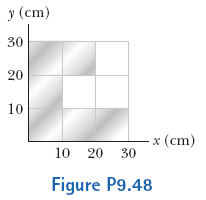# Problem: A uniform piece of sheet metal is shaped as shown in the figure. Compute the x and y coordinates of the center of mass of the piece.

###### FREE Expert Solution
92% (297 ratings)
###### Problem Details

A uniform piece of sheet metal is shaped as shown in the figure. Compute the x and y coordinates of the center of mass of the piece.What scientific concept do you need to know in order to solve this problem?

Our tutors have indicated that to solve this problem you will need to apply the Intro to Center of Mass concept. You can view video lessons to learn Intro to Center of Mass. Or if you need more Intro to Center of Mass practice, you can also practice Intro to Center of Mass practice problems.

How long does this problem take to solve?

Our expert Physics tutor, Julia took 3 minutes and 27 seconds to solve this problem. You can follow their steps in the video explanation above.

What professor is this problem relevant for?

Based on our data, we think this problem is relevant for Professor Velissaris' class at UCF.

What textbook is this problem found in?

Our data indicates that this problem or a close variation was asked in Physics for Scientists and Engineers - Serway Calc 9th Edition. You can also practice Physics for Scientists and Engineers - Serway Calc 9th Edition practice problems.